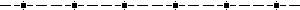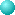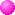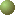=-=  Hypergraph Dualization Repository  =-=

Program Codes and Instances for Hypergraph Dualization (minimal hitting set enumeration)

Keisuke Murakami & Takeaki Uno (uno@nii.jp)A hypergraph F is a set family defined on vertex set V such that each set in F (called hyperedge) is a subset of V. For example, the set of {1,2}, {3}, {2,3,4} is a hypergraph. A hitting set H of F is a subset of V such that H intersects any hyperedge of F. A hitting set including no other hitting set is called minimal. For example, {1,3,4} is a hitting set of F, and {1,3} is a minimal hitting set. The dual of a hypergraph F is the set of all minimal hitting sets of F. The problem of computing the dual (also known as minimal hitting set enumeration, minimal set-covering enumeration) is one of a fundamental topic in computer science, and widely studied. For the evaluation of the algorithms and practical benefits, this page provides implementations of the algorithms including that by Takeaki Uno, and many problem instances used in the existing studies. All the instances and implementations are used in our study . We are welcome the submittion of the instances/implementations for the growth of this research field.

contact:  Takeaki Uno: uno@nii.ac.jp

ü@====  Implementations  ====

ü@SHD (Sparsity-based Hypergraph Dualization, ver. 3.1)   (C code, Takeaki Uno)     Explanation

This is an implementation coded by Takeaki Uno. In our experiments, this achieves the fastest for many instances among these implementations. Execution with "0" command is RS algorithm, and with "D" command is DFS algorithm.BEGK    (C code, code by Prof. Khaled Elbassioni, original page)    Note: the code is provided as is, with no warranty, we thank him for his permitting us to provide his code on our page.

A quasi polynomial time algorithm. In the sense of time complexity, fastest in this page.DL    (C code, Keisuke Murakami)

Breadth first search type algorithm. Add hyperedges one by one with updating the dual.BMR    (C code, Keisuke Murakami)

An improved version of DL algorithm. Add hyperedges in the lexicographic order.KS    (PASCAL, coded by Elias C. Stavropoulos, original page)

Depth-first search version of DL algorithm. Needs small memory space.  (p2c applied code is included, which needs p2c, and can be compiled with %gcc -o *** ***.c -I/var/tmp/p2c-root/usr/include -lp2c)MTminer    (C code, François Rioult, from this page)

A hill-climbing type algorithm, i.e., recursively adds vertices in increasing order. Needs much memory.

ü@====  Instances  ====

ü@ac (accidents): (all zip)   the complement of the set of maximal frequent itemsets with support threshold t, of dataset "accidnent" taken from FIMI repository for frequent itemset mining. A minimal hitting set corresponds to a minimal infrequent itemset. (a frequent itemset is a vertex subset included in at least t hyperedges, and a frequent itemset included in no other frequent itemset is called maximal). "ac_90k" means that the threshold is 90,000.bms (BMS-WebVeiw2): (all zip)   the complement of the set of maximal frequent itemsets with support threshold t, of dataset "BMS-WebView2" taken from FIMI repository for frequent itemset mining. A minimal hitting set corresponds to a minimal infrequent itemset. (a frequent itemset is a vertex subset included in at least t hyperedges, and a frequent itemset included in no other frequent itemset is called maximal). "bms2_100" means that the threshold is 100.connect-4 (win): (all zip)     the set of minimal winning borad of a board game "connect-4". The files are the first k hyperedges. This data is taken from UCI machine learning repository. "w200" means it containes the first 200 hyperedges.connect-4 (lose): (all zip)     the set of minimal winning borad of a board game "connect-4". The files are the first k hyperedges. This data is taken from UCI machine learning repository. "l200" means it containes the first 200 hyperedges.matching: (all zip)    matching of size k/2 (so, k vertices); the file is composed of hyperedges {1,2}, {3,4}, {5,6},...dual-matching: (all zip)    dual of the matching of size k/2.Threshold graph (TH): (all zip)  the hyperedge set of this series of hypergraphs is  { {i,j} | {1 <=  i, j <= n, j is even }.Self-Dual Threshold graph (SDTH): (all zip)    the hyperedge set of these graphs are {n,n-1}, each hyperedge of TH(n-2) + {n}, each hyperedge of TH(n-2) + {n-1}.Self-Dual Fano Plane graph (SDFP): (all zip)  the hyperedges are the union of k-disjoint copies of  {{1,2,3},{1,5,6},{1,7,4},{2,4,5},{2,6,7},{3,4,6},{3,5,7}}.uniform random: (all zip)     random instances; each vertex is included in a hyperedge in the probability p. (generated by Prof. Alain Bretto)

ü@====  Experiments  ====

ü@ac (accidents):   time [csv]   memory [csv]   #iterations [csv]   #minimality checks [csv]bms (BMS-WebVeiw2):   time [csv]   memory [csv]   #iterations [csv]   #minimality checks [csv]connect-4 (win):   time [csv]   memory [csv]   #iterations [csv]   #minimality checks [csv]connect-4 (lose):   time [csv]   memory [csv]   #iterations [csv]   #minimality checks [csv]matching:   time [csv]   memory [csv]   #iterations [csv]   #minimality checks [csv]dual-matching:   time [csv]   memory [csv]   #iterations [csv]   #minimality checks [csv]Threshold graph:   time [csv]   memory [csv]   #iterations [csv]   #minimality checks [csv]Self-Dual Threahold graph:   time [csv]   memory [csv]   #iterations [csv]   #minimality checks [csv]Self-Dual Fano Plane graph:   time [csv]   memory [csv]   #iterations [csv]   #minimality checks [csv]uniform random:   time [csv]   memory [csv]   #iterations [csv]   #minimality checks [csv]

ü@

----------------------------------

 Leonid Khachiyan, Endre Boros, Khaled M. Elbassioni, Vladimir Gurvich, "An Efficient Implementation of a Quasi-polynomial Algorithm for Generating Hypergraph Transversals and its Application in Joint Generation", Discrete Applied Mathematics 154(16): 2350-2372 (2006)

 G. Dong and J. Li, "Mining Border Descriptions of Emerging Patterns from Dataset Pairs", Knowledge and Information Systems 8: 178-202 (2005).

 J. Bailey, T. Manoukian, and K. Ramamohanarao, "A Fast Algorithm for Computing Hypergraph Transversals and its Application in Mining Emerging Patterns", 3rd IEEE International Conference on Data Mining (ICDM 2003):485-488 (2003).

 D. J. Kavvadias and E. C. Stavropoulos, "An Efficient Algorithm for the Transversal Hypergraph Generation", Journal of Graph Algorithms and Applications 9: 239-264 (2005).

 C. He'bert, A. Bretto and B. Cre'milleux, "A Data Mining Formalization to Improve Hypergraph Minimal Transversal Computation", Fundamental Informaticae 80:415-433 (2007).

 Keisuke Murakami, Takeaki Uno, "Efficient Algorithms for Dualizing Large Scale Hypergraphs",

ü@ü@topü@        ü@NII topü@